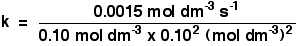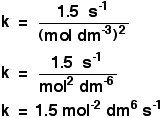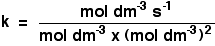Chemguide: Support for CIE A level Chemistry

```
```

Learning outcome 26: Reaction kinetics

26.1: Simple rate equations, orders of reaction and rate constants

```
```

Learning outcome 26.1.4

This statement is about calculating rate constants.

Before you go on, you should find and read the statement in your copy of the syllabus.

```
```

Finding a rate constant from initial rate experiments

In statement 26.1.2 we looked at finding the rate equation from initial rate data for two examples. But we didn't go on to calculate values for the rate constants.

Example 1

You were given the following data:

```
```
Experiment[A]
(mol dm-3)
[B]
(mol dm-3)
initial rate
(mol dm-3 s-1)
10.100.100.0015
20.200.100.0030
30.200.200.0120
```
```

That rate equation turned out to be:

Rate = k[A][B]2

Look at the numbers again to make sure that you can still do this!

You can now go on to use this to find a value for the rate constant, k. All you need to do is to substitute values for the rate and the concentrations into this equation and solve it for k.

Which experimental values do you use? It doesn't matter. Whichever you use will give the same value for k. It makes sense to use the easiest looking values, such as experiment 1, but it really doesn't matter. Prove it to yourself by doing the calculation three times if you can be bothered.

Taking values from experiment 1:

0.0015 = k x 0.10 x 0.102

Rearranging this to find k gives a value for k of 1.5

But you can't leave it there. It has to have some units. Unfortunately, the units vary depending on the overall order of the reaction, and you have to work them out each time.

There are two ways you can do this:

When you substitute the numbers into the equation, include all of their units as well. So in this instance, the expression for k would be:Then, as well as working out the numbers, you also cancel and rearrange the units. That gives:Personally, I find it slightly less confusing (and I am also slightly less likely to make mistakes) if I process the numbers and the units separately.

So I would do the calculation just with the numbers in the expression, and then repeat it writing the units instead of the numbers. The first line of working out the units would be:Then you just cancel and rearrange the units as above. In an exam, you wouldn't have to show how you worked out the units - you just have to get them right! It will be worth a mark, and you can't afford to waste one of those in a CIE exam.

Note:  I have explained this in much more detail on pages 129 - 130 of my chemistry calculations book, and with detailed working on later examples as well.

```
```

Example 2

Experiment[A]
(mol dm-3)
[B]
(mol dm-3)
initial rate
(mol dm-3 s-1)
10.120.160.0014
20.120.240.0021
30.150.320.0035
```
```

This time the rate equation turned out to be:

Rate = k[A][B]

By substituting numbers from any one experiment into this equation, you can go on to find the value of the rate constant (together with its units.)

If you work this out, you will find that k = 0.073 mol-1 dm3 s-1.

The answer is given to 2 significant figures because the numbers you are using to work it out are all to that degree of accuracy. Work this out for yourself (including the units) to be sure that you can do it.

```
```

The best way of learning to do these questions (as with all numerical problems) is to practise doing lots and lots of them.

```
```

Calculating rate constants from half-lives

This has already been covered in statement 26.1.3. Provided you know the equation, these calculations are trivial.

```
```

Go to the Section 26 Menu . . .